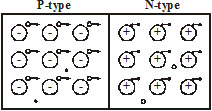## Pages

### Unit I.2 (E.E.)

(i) N-Type Semiconductors: -                                                     (AKTU - 2005-06, 07-08, 11-12)
An n-type semiconductor is created by introducing impurity elements that have five valencing impurity elements that have five valence electrons (pentavalent), such as antimony (Sb) arsenic (As) and phosphorus (P). If the amount of impurity is very small (say, one part in one million), we can assume that each impurity atom is surrounded, all around, by silicon atomsThere is, however, an additional fifth electron due to the impurity atom, which is unassociated with any particular covalent bond. This remaining electron, loosely bound to its parent (antimony) atom, is relatively free to move within the newly formed N-type semiconductor. Since the inserted impurity atom has donated a relatively “free” electron to the structure : diffused impurities with five valence electrons are called donor atoms.
(ii) P-Type Semiconductors: -                                                              (AKTU - 2005-06, 07-08,11-12)
If we add a trivalent impurity such as boron (B), aluminium (Al), gallium (Ga) or indium (In) to the intrinsic semiconductor, the result is a p-type semiconductor. Let us consider a sample of intrinsic (pure) silicon to which a very small amount of boron is added. The boron atom in the crystal has only three valence electrons. These electrons form covalent bonds with the three neighbouring silicon atoms. The fourth neighbouring silicon atom is unable to form a covalent bond with the boron atom because the boron atom does not have the fourth electron in its valence orbit.

Note that there is now an insufficient number of electrons to complete the covalent bonds. The resulting vacancy is called a hole, indicating the absence of electron. Since the resulting vacancy will accept a free electron : the  diffused impurities with three valence electrons are called acceptor atoms.
* Representation of N-type Semiconductors: -* Representation of P-type Semiconductors: -
o - Hole (Positive charge carrier)
Here they are majority carriers
·  Free electron (negative charge carrier)
Here they are minority carriers
immobile negative ion (acceptor)
* Effect of Temperature on Semiconductor: -
As the temperature rises, an increasing number of valence electrons absorb sufficient thermal energy to break the covalent bond and to contribute the number of free carriers. Therefore, semiconductor materials have a negative temperature coefficient of resistance.
Note: -
An intrinsic semiconductor (germanium or silicon) at absolute zero temperature, behaves as a perfect insulator.
* Effect of Temperature on Extrinsic Semiconductor: -
If we increase the temperature of an N-type semiconductor, since all the donors have already donated their free electrons (at room temperature), the additional thermal energy only serves to increase the thermally generated carriers. As a result, the concentration of minority carriers increase. Eventually, a temperature is reached when the number of covalent bonds that are broken is very large, so that the number of holes is approximately the same as the number of electrons. The extrinsic semiconductor now behaves essentially like an intrinsic semiconductor. This critical temperature is 85oC for Ge and 200oC for Si.
* Fermi Level: -
It is defined as the energy state or level which has a 50% probability of being filled by an electron.
* Fermi Level in Intrinsic Semiconductor: -
For intrinsic semiconductor, the fermi-level lie mid-way between conduction band and valence band. Shown in fig. (1).
* Fermi-Level in N-type Semiconductor: -
For N-type semiconductor, the number of electrons increases in the conduction band because of donor impurities. Due to this, the fermi level shifts upward closer to the conduction band. Shown in fig. (2)
*Fermi Level in P-type Semiconductor: -For a p-type semiconductor, the number of electrons decreases in the conduction band because of acceptor impurity. Due to this, the fermi-level is lowered and becomes closer to the valence band. Shown in fig. (3).
Semiconductor Diode / P-N Junction Diode: -
A P-N junction diode is an electronic device that has two electrodes (anode and cathode) and that allows current to flow in only one direction, and resisting current flow in the other direction.If we join a piece of p-type semiconductor to a piece of N-type semiconductor such that the crystal structure remains continuous at the boundary, a PN-junction is formed.
Note: -
A PN-junction can’t be made by simply pushing the two pieces together. Special fabrication techniques are needed to form a PN-junction.
Formation of Depletion Layer: -                                                                  (AKTU - 2010-11, 12 - 13)
• As we know, the P-region has more number of holes than N-region and N-region has more number of electrons than P-region. Due to this concentration gradient, holes from the P-region diffuse into the N-region, and combines with the electrons. Similarly the electrons from the N-region diffuse into the P region and combines with the holes.• After a few recombination of holes and electrons in the immediate neighbourhood of the junction, a restraining force is set up automatically. The force is called potential barrier.• Further holes trying to diffuse into the N-region are repelled by the uncovered

Next Page